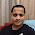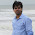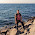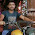## Saturday, June 7, 2014

### Find nth node from the end of a linked list in Java

In this post, we'll look into the different approaches of finding the nth node from the end of a linked list.

Let's have a code of linked list before discussing the different approaches:

ListNode
Linked List
This simply create the linked list for understanding approaches to find nth node from the end of a linked list
Note : n the element means counting start from 1 instead of 0 from end.

Approach 1 Brute-Force approach
In this method, start with the first node and count how many nodes are there after that node. If the number of node are < n then return saying "Fewer no. of nodes". If the number of nodes >n then go to next node. Continue this until the numbers of nodes after current nodes are n
Time Complexity : O(n^2), Space Complexity : O(1)

Approach 2 We can improve the complexity of above approach using HashMap. In this approach, create a hash map whose entries are <position of node, node address>. This means, key is the position of the node in the linked list and value is the address of the node.

By the time we traverse the complete list, we can find the list length. Let's say the list length is M. To find the nth from end of linked list, we can convert this to M-n th from the beginning. Since we already know the length of the list, it's just a matter of returning M-n key value from the hash map.
Time Complexity : O(n) for creating the HashMap, Space Complexity : O(n)

Approach 3 We can solve the above approach without creating a Hash Map. We are using Hash map to find the linked list size. We can find the size of the linked list just bye starting at the head node and traversing the list. So, we can find the length of the list without creating the Hash map. After find the length, compute M-n th and with one more scan we can get the M-n th node from the beginning. This solution need two scans : one for finding the length of linked list and other for finding M-n node from the beginning.
Time Complexity : Time for finding the length=Time for finding the M-n th node from the beginning. Therefore, T(n)=O(n)+O(n)=O(n), Space Complexity : O(1)

Approach 4 We'll solve this problem in one scan. In this approach, use two pointers pNthNode and pTemp. Initially, both points to the head node of the list. pNthNode  start moving only after pTemp made n moves. From there both moves forward until pTemp reaches end of the list. As a result pNthNode point to the nth node from the end of the linked list.
Time Complexity : O(n), Space Complexity : O(1)
Same approach can be use to delete nth element from a linked list.

If you know anyone who has started learning Java, why not help them out! Just share this post with them.
Thanks for studying today!...

#### 5 comments:

1.I like you post and this site they are really informative...... but problem is we cannot copy code from the page to test it . we have right every part of code. if you make up coming post copy-able we would feel happy.

1.I agree with you Ismail and even I don't have any problem in sharing the same code, but, I want that who ever read these codes should try by himself instead of just copy and paste.

2.yes okey I understood the intention.. thank you

2.Hi, Great.. Tutorial is just awesome..It is really helpful for a newbie like me.. I am a regular follower of your blog. Really very informative post you shared here. Kindly keep blogging. If anyone wants to become a Java developer learn from Java Training in Chennai. or learn thru Java Online Training from India . Nowadays Java has tons of job opportunities on various vertical industry.

3.